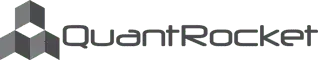Disclaimer

This notebook looks at how wide the typical spread is between different CL contracts.

First, load the last 30 days of prices. For the BID_ASK bar type, the Open field contains the average bid and the Close field contains the average ask:

Using a DataFrame of prices, we can use the function get_contract_nums_reindexed_like to obtain a similarly indexed DataFrame showing each contract's numerical sequence in the contract chain as of any given date. Using the limit parameter, we ask the function to sequence the next 15 contracts.

Next we get a Series of midpoints for each contract num by masking the midpoints DataFrame with the contract num DataFrame and taking the mean of each row. In taking the mean, we rely on the fact that the mask leaves only one non-null observation per row, thus the mean simply gives us that observation.

We loop through the contract months to generate a matrix of dollar spreads between contract months:

The matrix can be used to generate a heat map, which reveals that the closer the contract months, the tighter the spreads:

## Next Up¶

Part 3: Moonshot Strategy